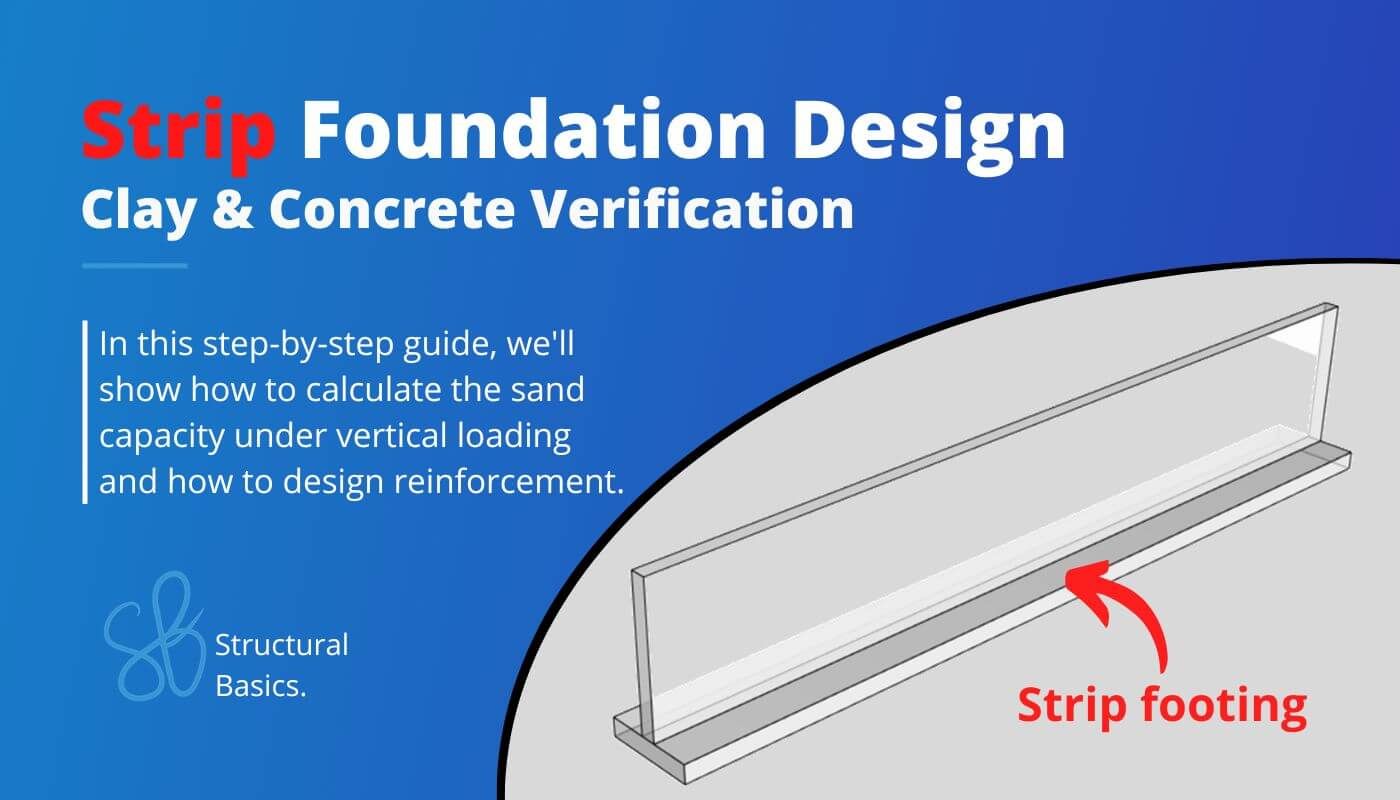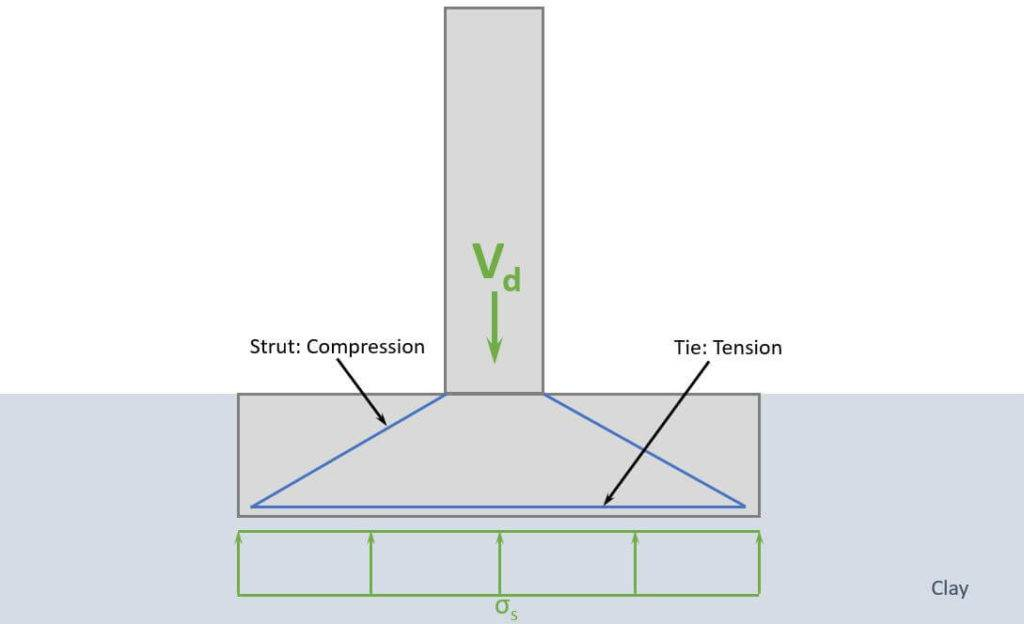# Strip Foundation In Clay {Design Of Soil And Concrete}Last updated: July 25th, 2023

Designing a strip foundation is never really taught fully in university or textbooks. 🙈🙈

It’s either the geotechnical or the reinforced concrete design.

But both are necessary for structural documentation. 📄📄

That’s why in this post we’ll show you, step-by step, how to design a strip foundation in clay with a worked example. We’ll cover both the geotechnical verification of clay according to Eurocode EN 1997-1 and the reinforced concrete verification according to Eurocode EN 1992-1-1.

Not much more talk, let’s dive into it. 🚀🚀

## 🙋‍♀️ What Is A Strip Foundation?

Strip foundations serve as a support system for walls in buildings. Typically, they consist of a concrete “block”, and in most cases steel reinforcement to resist tensile forces. These foundations are placed on soil and spread the concentrated load from the wall to a wider area of the soil.

Strip footings or foundations in general stand on sand, clay, rock, or a mix of 2 soil types. In this tutorial, we design a strip foundation which is embedded in clay.

Strip foundations usually have rectangular 🟦🟦 bases.

## 📃 Process of Strip Foundation Design

Before we dive into the nerdy calculations 😋😋, it’s good to get an overview of the steps that need to be taken to design a strip foundation, which is supported by clay and exposed to a vertical line load.

1. Calculate characteristic loads that act on strip foundation
3. Define properties of the sand and concrete
4. Geotechnical design – bearing resistance verification of clay
5. Reinforced concrete design – bending verification
6. Reinforced concrete design – shear verification

The loads of a structure depend on its location, geometry, building type and other factors.

We’ll assume in this tutorial that we design the strip foundation of a multi-storey building. 👇👇

Now to calculate the characteristic loads acting on the strip foundation, we need to know what loads are applied where and how the loads travel through the different elements and to the foundations.

In this simplified design guide, the following vertical loads are considered:

Now, in the structural design of such a building, a lot more loads are considered such as seismic and wind load and imperfections.

❗ The following load transfer explanation works if the building is a traditional structure with simply supported beams and columns❗

1. Live, snow and dead load (self-weight of roofing) are applied as area loads [kN/m2] on the roof. This could be precast hollow core slabs, which transfer the loads to beams and walls.
2. The reaction force of a slab is then the characteristic vertical load that is applied to the wall.
3. We do that for every floor, and the reaction forces are added up and applied to the strip foundation.

The following characteristic load values are assumptions.

❗❗ The characteristic values of loads depend on a lot of different factors like location, National Annex and geometry of the building and roof to name just a few. Loads therefore need to be calculated for every structure. ❗❗

You can also use our load combination generator, which creates the load combinations automatically for you, and you can copy & paste the table into Word. 🔥🔥

So the vertical design load we are designing the pad foundation for is:

$$V_{d} = 315 kN/m$$

## 🟫 Geotechnical Soil Properties

The strip foundation is embedded into the soil. In our example, it’s clay.

For this tutorial, we use the following soil properties.

## ⬜ Concrete and Reinforcement Properties

For this tutorial, we use the following concrete and reinforcement properties.

## 🕵️‍♂️ Geometry Of The Foundation And Wall

Now, in countries, where the temperatures can get low, it’s common to have a minimum depth, where frost can’t affect the groundwater any more. Because if it does, it can lift up the foundation.

In Denmark, for example, 0.9 m is a common minimum depth.

## ✍️ Geotechnical Design – Bearing Resistance (EN 1997-1)

As we only have a vertical load acting on the pad foundation, we only have to verify that the design bearing resistance of the clay is greater than the design load. Let’s do that. 👍👍

For the final formula, we first need to calculate a lot of factors.

Shape factors (EN 1997-1 (D.1))

$$s_c = 1 + 0.2 \dot \frac{w}{l} = 1.0$$

Inclination of loads (EN 1997-1 (D.1))

$$i_c = \frac{1}{2} \dot (1 + \sqrt{1 – \frac{H_d}{w \cdot l \cdot c_{u.d}}}) = 1$$

Inclination of the foundation base (EN 1997-1 (D.1))

$$b_c = 1 – 2 \cdot \frac{\alpha}{\pi + 2} = 1$$

Effective vertical stresses

$$q = \gamma ‘ \cdot h’ = 4 kN/m^2$$

Design bearing resistance (EN 1997-1 (D.1))

$$\frac{R}{A} = (\pi + 2) \cdot c_{ud} \cdot b_c \cdot s_c \cdot i_c + q = 261.1 kN/m^2$$

$$R_d = 261.1 kN/m^2 \cdot w = 365.5 kN/m$$

Utilization

$$\eta = \frac{V_d}{R_d} = \frac{322.7 kN/m}{365.5 kN/m} = 0.88$$

Therefore, the bearing capacity of the soil (clay) is verified. 👍👍

## 👨‍🔬 Reinforced Concrete Design – Bending Verification

A strut and tie model is used to transfer the line load to the soil and spread the load equally along the foundation base.

Now, as concrete can’t take much tension, reinforcement will be used for the tie, and we are going to calculate how much.

The compression zone of the strip foundation creates pressure in the soil, which is visualized in the next picture.Earth pressure $$\sigma$$ equals vertical line load $$V_d$$.

$$\sigma_s = \frac{V_d}{w} = 230.5 kN/m^2$$

The soil pressure $\sigma_s$ creates a bending moment in the pad foundation, and we use a cantilever beam to calculate that bending moment.

The length of the cantilever is $l_c = w/2 – w_c/2 = 0.55 m$

Now, the bending moment can be calculated.

$$M_{Ed} = \sigma_s \cdot 1m \cdot l_c \cdot l_c/2 = 230.5 kN/m^2 \cdot 1.4 m \cdot 0.55 m \cdot \frac{0.55 m}{2} = 34.9 kNm$$

We set the diameter of the rebars to $d_s = 10 mm$.

The lever arm of the upper layer of reinforcement is calculated as

$$d = h – c – d_s – \frac{d_s}{2} = 0.43 m$$

The required reinforcement is calculated with the following formulas. 👇👇

$$\mu = \frac{M_{Ed}}{1m \cdot d^2 \cdot \eta \cdot f_{c.d}} = 0.011$$

$$\omega = 1 – \sqrt{1 – 2 \cdot \mu} = 0.011$$

$$A_{s.req} = \omega \cdot \frac{1m \cdot d \cdot \eta \cdot f_{c.d}}{f_{y.d}} = 175.8 mm^2$$

Check of minimum reinforcement according to EN 1992-1-1 9.2.1.1 (9.1N).

$$A_{s.min} = max(0.26 \cdot \frac{f_{ctm}}{f_{y.k}} \cdot 1m \cdot d; 0.0013 \cdot 1m \cdot d) = 565.5 mm^2$$

Because $A_{s.min} > A_s$, $A_{s.min}$ determines the reinforcement used.

Cross-sectional area of a rebar with $d_s = 10 mm$.

$$A_{s.1} = \pi \cdot (\frac{d_s}{2})^2 = 78.5 mm^2$$

Amount of rebars:

$$n = roundup(\frac{A_{s.min}}{A_{s.1}}) = 8$$

Reinforcement area:

$$A_s = n \cdot A_{s.1} = 628.3 mm^2 > A_{s.min}$$

Now, as the minimum reinforcement determined the reinforcement, we apply the same reinforcement to the longitudinal direction.

## 👩‍🏫 Reinforced Concrete Design – Shear Verification

First we check if shear reinforcement is required according to EN 1992-1-1 6.2.2.

### Members not requiring design shear reinforcement

EN 1992-1-1 6.2.2 (1)

$$k = 1 + \sqrt{\frac{200}{\frac{d}{mm}}} = 1.68$$

$$\rho_1 = min(\frac{A_s}{w \cdot d}; 0.02) = 0.001$$

Design value of the shear resistance (EN 1992-1-1 (6.2.a))

$$\upsilon_{Rd.c} = max(\frac{0.18}{\gamma_c} \cdot k \cdot (100 \rho_1 \cdot f_{c.k})^{\frac{1}{3}}; \frac{0.051}{\gamma_c} \cdot k^{\frac{3}{2}} \cdot \sqrt{f_{c.k}}) = 0.38 MPa$$

$$V_{Rd.c} = \upsilon_{Rd.c} \frac{\cdot w \cdot d}{1m} = 232.8 kN/m$$

Shear reinforcement required because $V_d > V_{Rd.c}$.

### Members requiring design shear reinforcement

EN 1992-1-1 Figure 6.5

$$z = 0.9 \cdot d = 0.39m$$

Coefficient taking into account the state of the stress in the compression chord:

$$\alpha_{cw} = 1.0$$

Strength reduction factor for concrete cracked in shear (EN 1992-1-1 (6.9))

$$\upsilon_1 = 0.6$$

$$cot(\theta) = 2.5$$

$$tan(\theta) = 0.4$$

Shear resistance (EN 1992-1-1 (6.9))

$$V_{Rd.max} = \alpha_{cw} \cdot w \cdot z \cdot \upsilon_1 \cdot \frac{f_{c.d}}{cot(\theta) + tan(\theta)} = 1955 kN$$

Verification is fulfilled. 👇👇

$$V_d < V_{Rd.max} = 1$$

Reduction of the design yield strength of the reinforcement (EN 1992-1-1 (6.8))

$$f_{ywd} = 0.8 \cdot f_{y.k} = 440 MPa$$

$$A_{sw} = \frac{V_d \cdot 1m}{z \cdot f_{ywd} \cdot cot(\theta)} = 749.3 \frac{mm^2}{m}$$

Inclination of the shear reinforcement

$$\alpha = 90 deg$$

Maximum spacing (EN 1992-1-1 (9.6N))

$$s_{l.max} = 0.75 \cdot d \cdot (1 + cot(\alpha)) = 0.326 m$$

We set the spacing of the bars to s = 250 mm.

Shear reinforcement required for the whole width:

$$A_{sw} = A_{sw} \cdot w = 1049 mm^2$$

Amount of bars:

$$n = roundup(\frac{w}{s}) = 6$$

Required cross-sectional area for one stirrup:

$$A_{sw.req} = \frac{A_{sw}}{2 \cdot n} = 87.4 mm^2$$

Required bar diameter:

$$d_{s.req} = \frac{2 \cdot \sqrt{A_{sw.req}}}{\pi} = 6 mm$$

Therefore, a stirrup diameter of $d_{s.s} = 10 mm$ is picked.

## 🙌 Conclusion

Once the bearing capacity of the clay, longitudinal and shear reinforcement of the strip foundation are verified, we successfully designed the strip foundation. 💯💯

If you are new to structural design, then check out more of our design tutorials where you can also learn how to design wood elements such as

But now, I would like to hear from you: Have you already designed a strip foundation in university or at your work? And which semester was that in? Tell us a bit about the structure it was supporting, as we all want to learn from each other. ✍️✍️

## 🙋‍♂️ Strip Foundation On Clay FAQ

What structural verifications do I have to do for a strip foundation in clay?

For only vertical loading, you have to verify the following 3 things:
– Bearing resistance of clay > Vertical design load
– Design the bending reinforcement
– Design the shear reinforcement

Which standards do I use to design a strip foundation in clay?

For the geotechnical design Eurocode 7 (EN 1997-1) is used if you are in Europe. For the concrete and reinforcement design Eurocode 2 (EN 1992-1-1) applies.